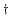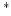Next: Random phases vs Gaussian

# Noisy spectra, long correlations, and intermittency in wave turbulence Phys. Rev. E 69, 066608 (2004)

Yuri V. Lvovand Sergey NazarenkoDepartment of Mathematical Sciences, Rensselaer Polytechnic Institute, Troy, NY 12180Mathematics Institute, The University of Warwick, Coventry, CV4-7AL, UK

04.30.Nk , 24.60.-k, 52.35.Ra, 92.10.Cg, 87.15.Ya

### Abstract:

We study the k-space fluctuations of the waveaction about its mean spectrum in the turbulence of dispersive waves. We use a minimal model based on the Random Phase Approximation (RPA) and derive evolution equations for the arbitrary-order one-point moments of the wave intensity in the wavenumber space. The first equation in this series is the familiar Kinetic Equation for the mean waveaction spectrum, whereas the second and higher equations describe the fluctuations about this mean spectrum. The fluctuations exhibit a nontrivial dynamics if some long coordinate-space correlations are present in the system, as it is the case in typical numerical and laboratory experiments. Without such long-range correlations, the fluctuations are trivially fixed at their Gaussian values and cannot evolve even if the wavefield itself is non-Gaussian in the coordinate space. Unlike the previous approaches based on smooth initial k-space cumulants, our approach works even for extreme cases where the k-space fluctuations are absent or very large and intermittent. We show, however, that whenever turbulence approaches a stationary state, all the moments approach the Gaussian values.

The concept of Wave Turbulence (WT), which describes an ensemble of weakly interacting dispersive waves, significantly enhanced our understanding of the spectral energy transfer in complex systems like the ocean, the atmosphere, or in plasmas [1,2,3,4,5]. This theory also became a subject of renewed interest recently, (see, e.g. [6,7,8,9]). Traditionally, WT theory deals with derivation and solutions of the Kinetic Equation (KE) for the mean waveaction spectrum (see e.g. ). However, all experimentally or numerically obtained spectra are noisy'', i.e. exhibit k-space fluctuations which contain a complimentary to the mean spectra information. Such fluctuations will be studied (for the first time) in this manuscript.Next: Random phases vs Gaussian
Dr Yuri V Lvov 2007-04-11